# Cosine Product Integration

I’m back from break, and we’ll go a little out of order at first since this is the last problem that’s similar to the ones we’ve been working on.

The problem: Cosine Product Integration.  This is problem AN14 in the appendix.

The description: Given a sequence of n integers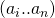, does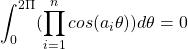?

Example:

Suppose our sequence was (1,2,3).  Then our integral is:This is just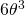, which integrates to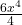, which over the interval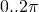is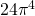Reduction: This one is in Plaisted’s 1976 paper. In it, he notes that if you look at the function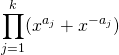the constant term in the power series expansion of that product is 0 if and only if the cosine integral is 0.  I have trouble seeing that myself.  The cooler thing is that you can make that constant term 0 if and only if you can take the sequence of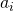elements and partition them into 2 sets with the same sum.  So we can take an instance of the Partition problem, use the elements of the set as the elements of our sequence and then feed them into the product formulas.

Difficulty: It really depends on how easily you can see the connection between the cosine integral and the product formula (and, of course, how easily you could have thought of it). I find it hard, so I’m giving it an 8.